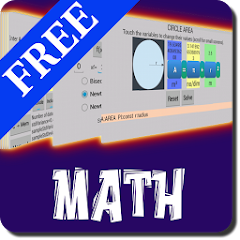# Interactive Math

1K+Everyone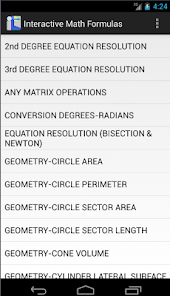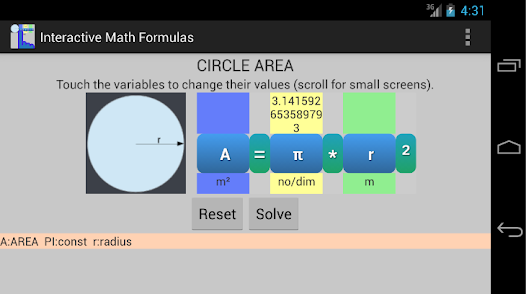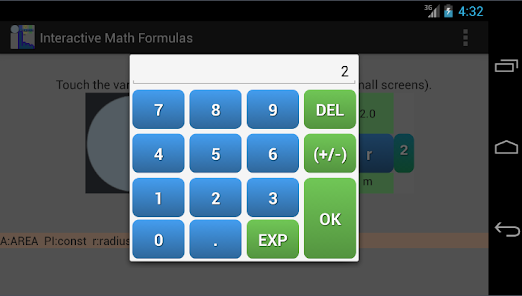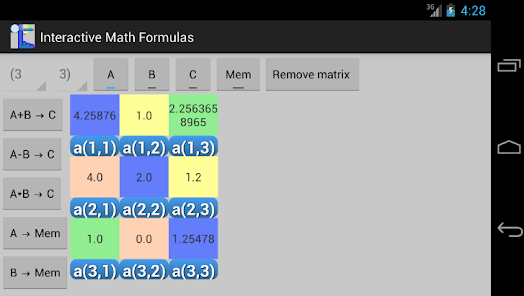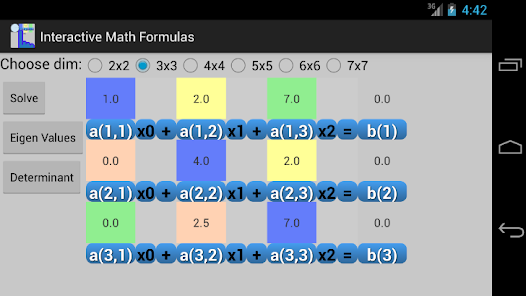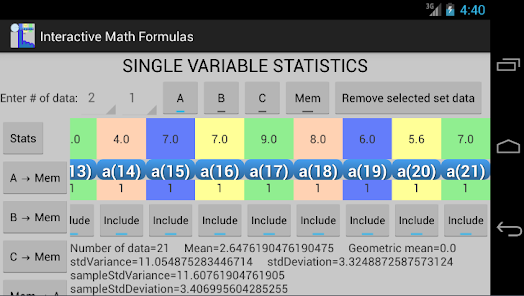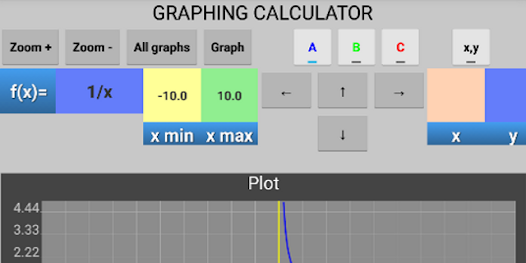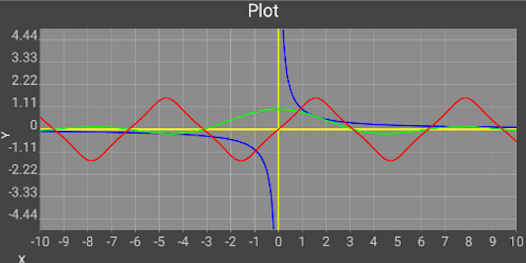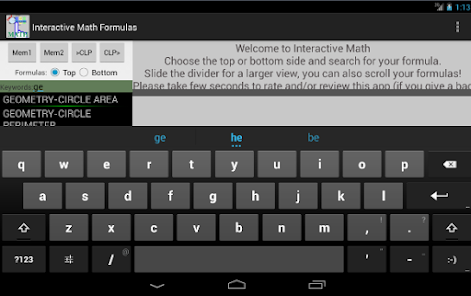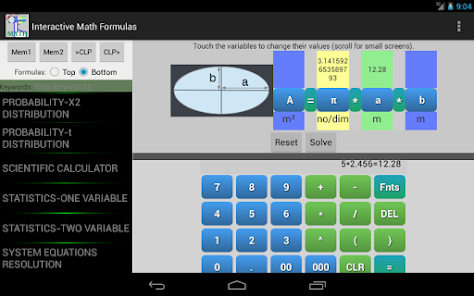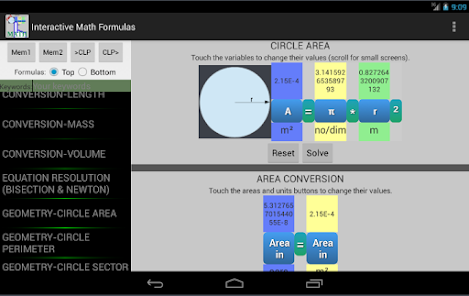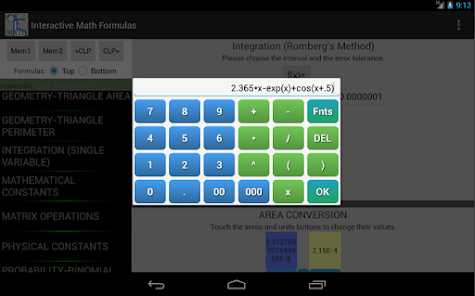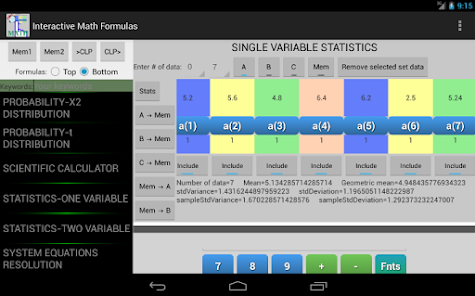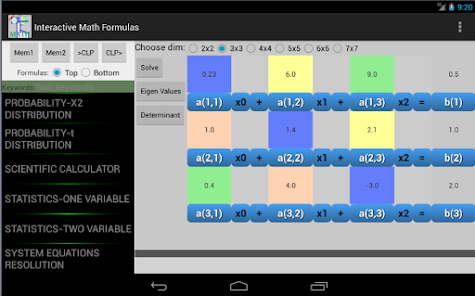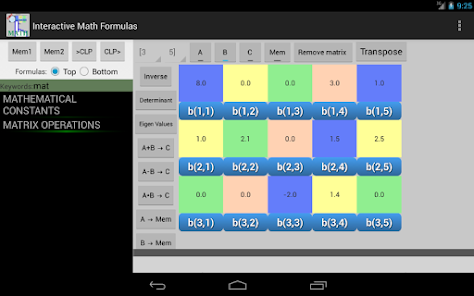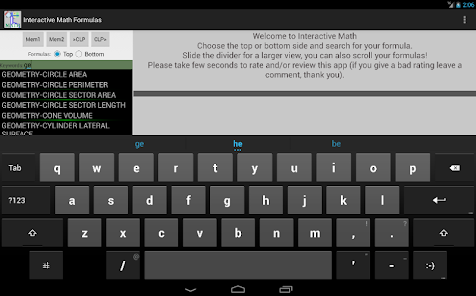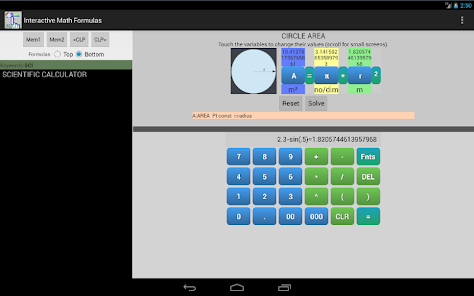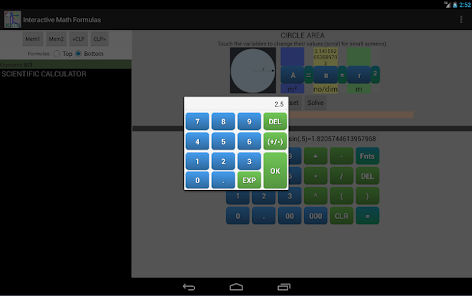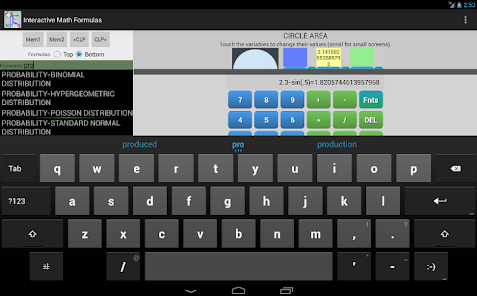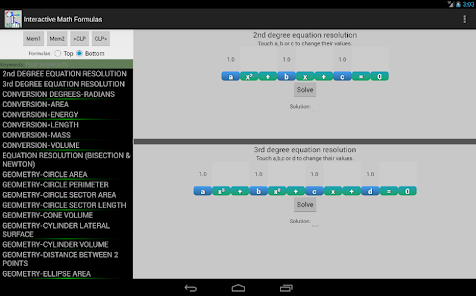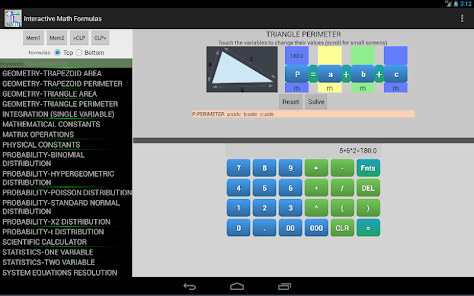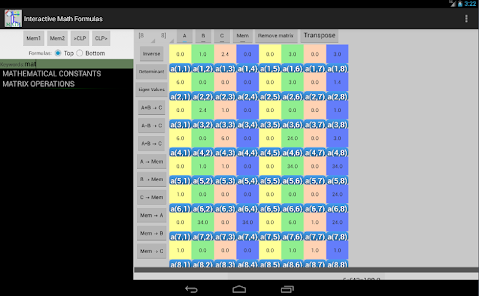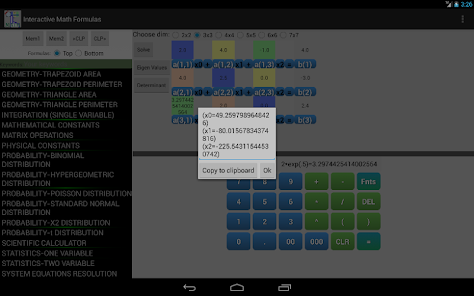This is a free version of "Interactive Math PRO", if you want the ad free version search in the android store for "Interactive Math PRO".
This app and math solver is ideal for students and graduates, you will find a variety of useful formulas and tools for your daily calculus, you can enter a value by touch and solve any variable of the equation, the interactive feature of the formulas make it easy to avoid typing or human errors.
The interface is made in a way that you can see above any variable it's value.

TOPICS:
"GEOMETRY-RECTANGLE PERIMETER",
"GEOMETRY-RECTANGLE AREA",
"GEOMETRY-TRIANGLE PERIMETER",
"GEOMETRY-TRIANGLE AREA",
"GEOMETRY-PARALLELOGRAM PERIMETER",
"GEOMETRY-PARALLELOGRAM AREA",
"GEOMETRY-TRAPEZOID PERIMETER",
"GEOMETRY-TRAPEZOID AREA",
"GEOMETRY-CIRCLE PERIMETER",
"GEOMETRY-CIRCLE AREA",
"GEOMETRY-CIRCLE SECTOR LENGTH",
"GEOMETRY-CIRCLE SECTOR AREA",
"GEOMETRY-ELLIPSE AREA",
"GEOMETRY-RECTANGULAR PARALLELEPIPED VOLUME",
"GEOMETRY-SPHERE SURFACE",
"GEOMETRY-SPHERE VOLUME",
"GEOMETRY-CYLINDER LATERAL SURFACE",
"GEOMETRY-CYLINDER VOLUME",
"GEOMETRY-CONE VOLUME",
"GEOMETRY-PYRAMID VOLUME",
"GEOMETRY-ELLIPSOID VOLUME",
"GEOMETRY-DISTANCE BETWEEN 2 POINTS",
"GEOMETRY-SLOPE BETWEEN 2 POINTS",
"GEOMETRY-RECTANGULAR-POLAR COORDINATES CONVERSION",
"SQUARE MATRIX OPERATIONS",
"SYSTEM EQUATIONS RESOLUTION",
"ANY MATRIX OPERATIONS",
"PROBABILITY-BINOMIAL DISTRIBUTION",
"2nd DEGREE EQUATION RESOLUTION",
"3rd DEGREE EQUATION RESOLUTION",
"EQUATION RESOLUTION (BISECTION & NEWTON)",
"PROBABILITY-HYPERGEOMETRIC DISTRIBUTION",
"INTEGRATION (SINGLE VARIABLE)",
"PROBABILITY-POISSON DISTRIBUTION",
"PROBABILITY-STANDARD NORMAL DISTRIBUTION",
"PROBABILITY-t DISTRIBUTION",
"PROBABILITY-X2 DISTRIBUTION",
"STATISTICS ONE VARIABLE",
"STATISTICS TWO VARIABLES",
"GEOMETRY-PYTHAGOREAN THEOREM",